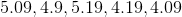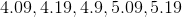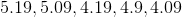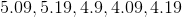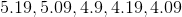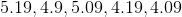## Example Questions

### Example Question #1 : How To Order Decimals From Least To Greatest Or From Greatest To Least

Put the following in order of least to greatest: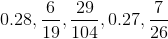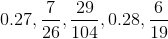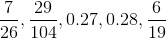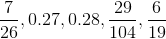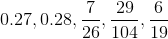Explanation:

Begin by converting each fraction into a decimal: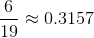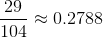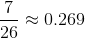Now we can put the decimals in order:

0.269, 0.27, 0.2788, 0.28, 0.3157

or: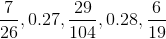### Example Question #1 : How To Order Decimals From Least To Greatest Or From Greatest To Least

Put these decimals in order from least to greatest: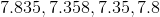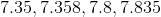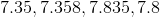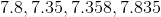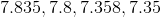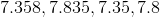Explanation:

To put these numbers in order from least to greatest we start by looking at the number before the decimal. In this case they're all the same (7) so we move to the first number to the right of the decimal. Since 8 is greater than 3 we know that 7.8 and 7.835 must be greater than the other two options. To decide if 7.8 or 7.835 is greater we look at the next number to the right. Since 7.8 doesn't have another number to the right we know that the number to the right must be 0. Since 3 is greater than 0 we know that 7.835 is greater than 7.8. We follow the same process to find that 7.35 is less than 7.358. Then we can put the numbers in order from least to greatest: 7.35, 7.358, 7.8, 7.835.

### Example Question #2 : How To Order Decimals From Least To Greatest Or From Greatest To Least

Order these decimals from greatest to least: